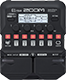# police

Discussion in 'Zoom G1/G1X Four' started by astrocarlo, Apr 7, 2022.

1. policeDevice: Zoom G1 Four
Firmware: 2.00

Name on device: police
Optimized for: Guitar Amp

Effects chain:every breath you take

Effect: "NoiseGate" (Dynamics), active - "yes"
"Detect" = EFXIN
"Depth" = 75
"Threshold" = 35
"Decay" = 20

Effect: "Gt GEQ 7" (Filter), active - "yes"
"100" = -3.0
"200" = -9.0
"400" = -10.0
"800" = -3.0
"1.6k" = 0.0
"3.2k" = -11.5
"6.4k" = 0.0
"VOL" = 48

Effect: "SuperCho" (Modulation), active - "yes"
"Depth" = 70
"Rate" = 50
"Tone" = 60
"Mix" = 42

Effect: "Delay" (Delay), active - "yes"
"Time" = 64
"F.B." = 21
"Mix" = 85
"Tail" = Off

Effect: "GateRev" (Reverb), active - "yes"
"Color" = 1
"Decay" = 55
"Tone" = 50
"Balance" = 60

Patch Volume: 100